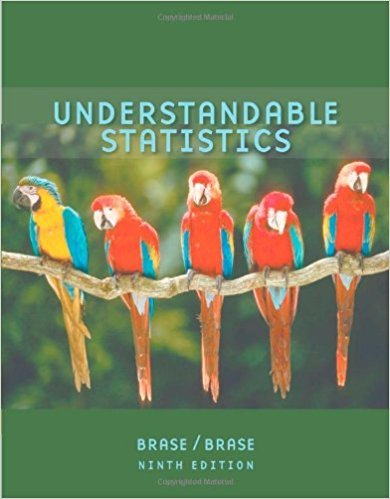×
Get Full Access to Understandable Statistics - 9 Edition - Chapter 9.1 - Problem 13
Get Full Access to Understandable Statistics - 9 Edition - Chapter 9.1 - Problem 13

×

# Insurance: Hail Damage Nationally, about 11% of the totalISBN: 9780618949922 213

## Solution for problem 13 Chapter 9.1

Understandable Statistics | 9th Edition

• Textbook Solutions
• 2901 Step-by-step solutions solved by professors and subject experts
• Get 24/7 help from StudySoup virtual teaching assistantsUnderstandable Statistics | 9th Edition

4 5 1 337 Reviews
17
5
Problem 13

Insurance: Hail Damage Nationally, about 11% of the total U.S. wheat crop is destroyed each year by hail (Reference: Agricultural Statistics, U.S. Department of Agriculture). An insurance company is studying wheat hail damage claims in Weld County, Colorado. A random sample of 16 claims in Weld County gave the following data (% wheat crop lost to hail). 15 8 9 11 12 20 14 11 7 10 24 20 13 9 12 5 The sample mean is Let x be a random variable that represents the percentage of wheat crop in Weld County lost to hail. Assume that x has a normal distribution and Do these data indicate that the percentage of wheat crop lost to hail in Weld

Step-by-Step Solution:
Step 1 of 3

Stats 401 Week 2 Relative frequency = How many times appeared in category ÷ Total items in category (like finding percent without multiplying by 100 at the end) For the median, if there is an even amount of number given then add the two middle terms then divide by 2. EX: 1 2 3 4 5 6. The two middle terms are 3 and 4 so 3 + 4 = 7. Then 7/2 = 3.5 (finding the average of those numbers). If there isn’t a mode (1 2 3 4 5 6) then write “NO MODE” not “The mode is zero” because that implies that there is the number 0 and that is what appears the most. Peak of curve is in the middle When the mean = median = mode the distribution is bell shaped or symmetric. Median > mean then it is left skewed Med

Step 2 of 3

Step 3 of 3

##### ISBN: 9780618949922

Unlock Textbook Solution